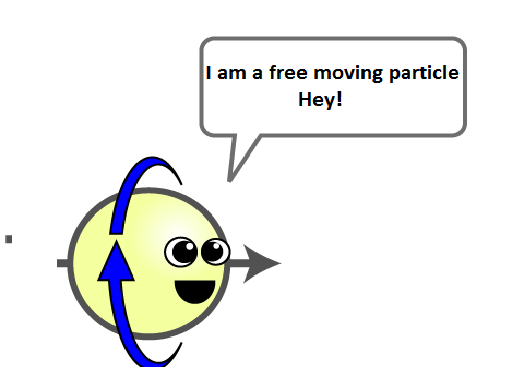# I am a free Particle!I am Kai and I am a free particle as my creator has named me.Kai a particle is moving in $2d$ motion.The particle's position is given by $x=4t^2+2t+3$ $y=2t+8$ Calculate the velocity of particle at t=3 seconds (round your answers to nearest hundredths)

×